Home | | Business Maths 12th Std | Homogeneous Differential Equations

# Homogeneous Differential Equations

Method of solving first order Homogeneous differential equation

Homogeneous Differential Equations

A differential equation of the form dy/dx = f (x, y)/ g (x, y) is called homogeneous differential equation if f (x, y) and g(x, y) are homogeneous functions of the same degree in x and y. (or)

Homogeneous differential can be written as dy/dx = F(y/x).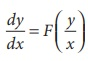## Method of solving first order Homogeneous differential equation

Check f ( x, y) and g ( x, y) are homogeneous functions of same degree.The given differential equation becomes v x dv/dx =F(v)

Separating the variables, we getBy integrating we get the solution in terms of v and x.

Replacing v by y/x  we get the solution.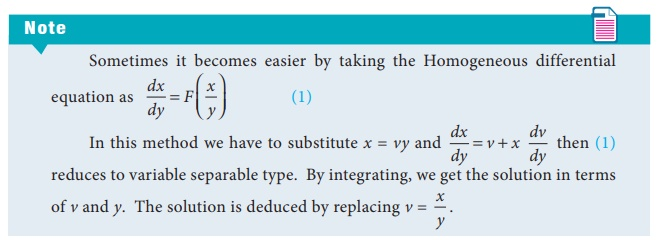Example 4.15

Solve the differential equation y2 dx + ( xy + x2 )dy = 0

Solution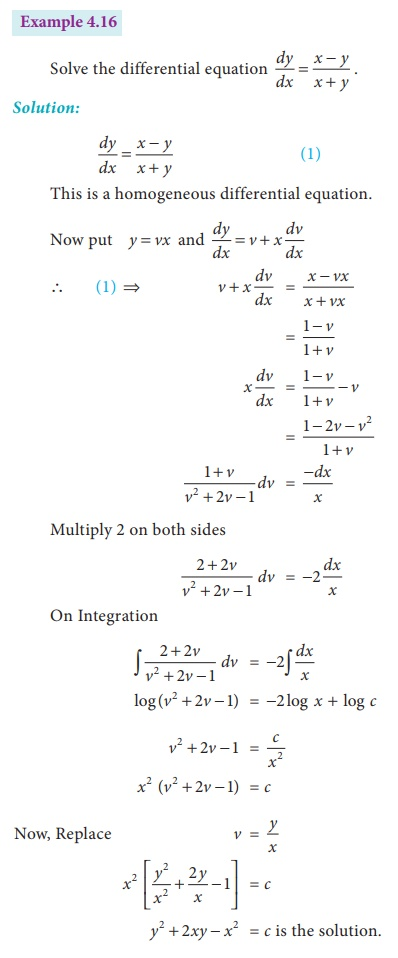Example 4.17

Find the particular solution of the differential equation x 2 dy + y ( x + y) dx = 0 given that x = 1, y = 1

Solution: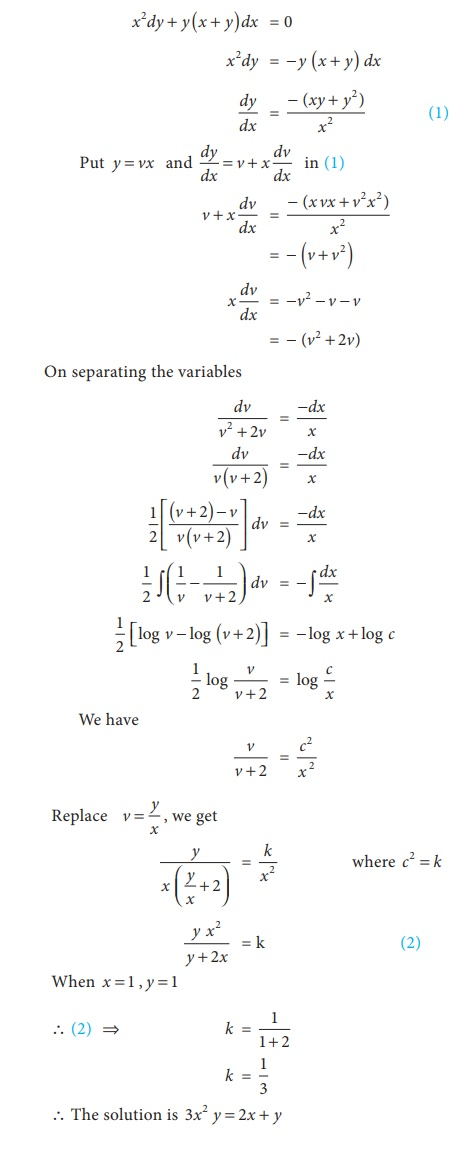Example 4.18

If the marginal cost of producing x shoes is given by (3xy + y2 ) dx + (x 2 + xy) dy = 0 and the total cost of producing a pair of shoes is given by ₹12. Then find the total cost function.

Solution:

Given marginal cost function is (x2 + xy) dy + (3xy + y2)dx=0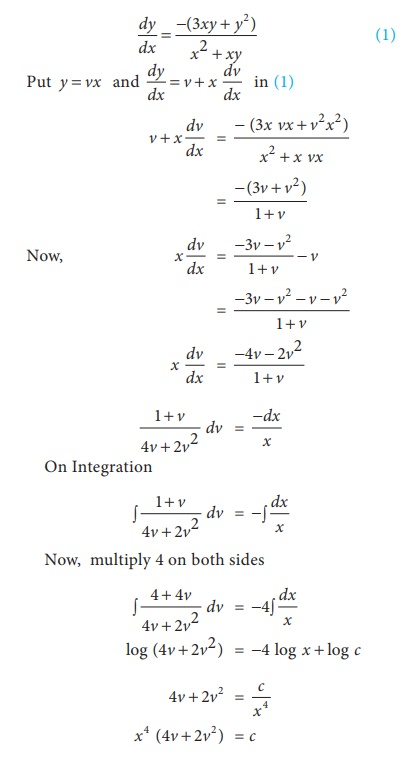Example 4.19

The marginal revenue ‘y’ of output ‘q’ is given by the equation. Find the total Revenue function when output is 1 unit and Revenue is ₹5.

Solution:Tags : Example Solved Problems with Answer, Solution, Formula , 12th Business Maths and Statistics : Chapter 4 : Differential Equations
Study Material, Lecturing Notes, Assignment, Reference, Wiki description explanation, brief detail
12th Business Maths and Statistics : Chapter 4 : Differential Equations : Homogeneous Differential Equations | Example Solved Problems with Answer, Solution, Formula